# CBSE Class 10 Science Sample Paper 2023 Set B

Read and download PDF of CBSE Class 10 Science Sample Paper 2023 Set B designed as per the latest curriculum and examination pattern for Class 10 issued by CBSE, NCERT and KVS. The latest Class 10 Science Sample Papers have been provided with solutions so that the students can solve these practice papers and then compare their answers. This will help them to identify mistakes and improvement areas in Science Standard 10 which they need to study more to get better marks in Grade 10 exams. After solving these guess papers also refer to solved Class 10 Science Question Papers available on our website to build strong understanding of the subject

## Sample Paper for Class 10 Science 2023 Pdf

Students can refer to the below Class 10 Science Sample Paper designed to help students understand the pattern of questions that will be asked in Grade 10 exams. Please download CBSE Class 10 Science Sample Paper 2023 Set B

### Science Class 10 Sample Paper 2023

SECTION – A

1. The diagram shows the reaction between metal and dil. acid. What is the reason for different behaviour of Mg in test tube B?(a) Mg is lighter element than dil. HCI.
(b) Mg reacts with dil. HC1 to produce H2 gas which helps in floating.
(c) Mg reacts with dil. HC1 to produce N2 gas which helps in floating.
(d) Mg reacts with dil. HCI to produce CO2 gas which helps in floating.

2. What is the difference in the molecular mass of any two adjacent homologues?
(a) 14 amu
(b) 15 amu
(c) 16 amu
(d) 17 amu

3. What is the focal length of a plane mirror?
(a) Infinity
(b) Zero
(c) 1
(d) + 1

4. The diagram below shows a leaf that was covered by a piece of black paper for a period of 3 days. After 3 days, the paper was removed. On testing, it was found that the area under the black paper tested negative for starch and the rest tested positive for starch. What was the experiment trying to test?
(a) If plants make their own food
(b) If light is required for plants to make food
(c) If plants can respire in the absence of light
(d) If plants can survive even in the absence of light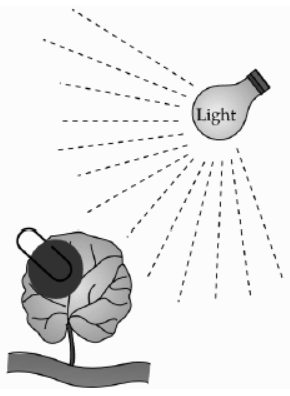5. A current-carrying conductor is held as shown: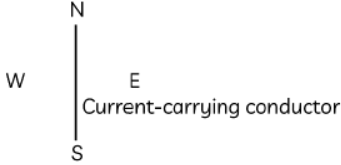In which direction should current be passed in the conductor in order to produce a clockwise magnetic field around the conductor?
(a) North to South
(b) South to North
(c) East to West
(d) West to East

6. For a current in a long straight solenoid N-pole and S-pole are created at the two ends. Among the following statements, the incorrect statement is:
(a) The field lines inside the solenoid are in the form of straight lines which indicates that the magnetic field is the same at all points inside the solenoid.
(b) The strong magnetic field produced inside the solenoid can be used to magnetise a piece of magnetic material like soft iron, when placed inside the coil.
(c) The pattern of the magnetic field associated with the solenoid is different from the pattern of the magnetic field around a bar magnet.
(d) The N-pole and S-pole exchange position when the direction of current through the solenoid is reversed.

7. At the time of short circuit, the electric current in the circuit:
(a) vary continuously
(b) does not change
(c) reduces substantially
(d) increases heavily

8. The graph below shows the variation of force acting on a conductor with current: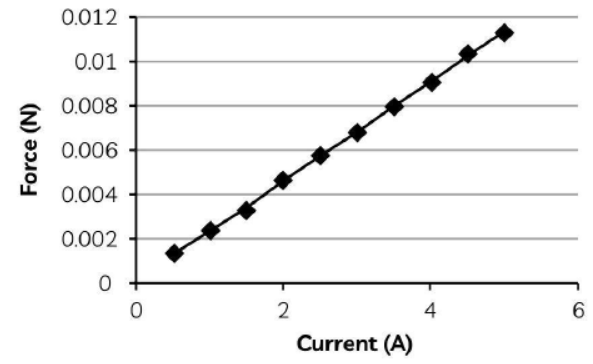After analyzing the graph, a student noted the following. Select the correct statement:
(a) The force acting on a conductor increases exponentially with increase in current.
(b) The force acting on a conductor decreases exponentially with increase in current.
(c) The force acting on a conductor increases linearly with increase in current.
(d) The force acting on a conductor decreases linearly with increase in current.

9. In a study it was found that fused ear lobes were found in more numbers within a population rather than free ear lobes. What can you infer from the above observation with respect to dominant/ recessive trait?
(a) Fused ear lobes – dominant
(b) Free ear lobes – dominant
(c) Fused ear lobes – recessive
(d) Both are dominant

10. What is the minimum resistance which can be made using the following resistors?(a) 1 Ω
(b) 2 Ω
(c) 4 Ω
(d) 3 Ω

11. In the given diagram, when the magnet is pushed into the solenoid, the pointer of the galvanometer deflects slightly to the left. Which of the following changes would cause the pointer to deflect through a larger angle?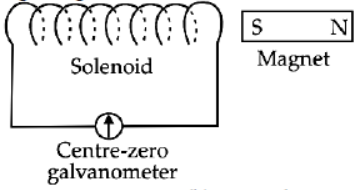(a) Move the magnet faster.
(b) Move the magnet away from the solenoid.
(c) Unwind some of the turns of the solenoid.
(d) Keep the magnet stationary.

12. Manish’s mother was baking cake in the kitchen. When Manish came back from school, he detected smell of hot cake from the drawing room. Why?
(a) Due to the presence of olfactory receptors in forebrain
(b) Due to the presence of taste buds
(c) Due to the presence of olfactory receptors in midbrain
(d) Due to the presence of olfactory receptors in hindbrain

13. Which of the following is not the role of decomposers in the ecosystem?
(a) They clean the environment.
(c) They participate in food chain.
(d) They replenish the nutrients in the soil.

14. In the given reaction : ZnO + C → Zn + CO.
I. ZnO is being oxidised.
II. CO is being reduced.
III. C is being oxidised.
IV. ZnO is being reduced.
Choose the correct statement.
(a) I and II only
(b) III and IV only
(c) I, II, and III only
(d) All of these

15. Common salt besides being used in kitchen can also be used as the raw material for making :
(i) washing soda (ii) bleaching powder (iii) baking soda (iv) slaked lime
(a) (i) and (ii)
(b) (i), (ii) and (iv)
(c) (i) and (iii)
(d) (i), (iii) and (iv)

16. A straight wire is placed between two poles of a magnet as shown in figure. If an alternating current passing through a wire then wire will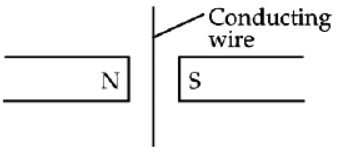(a) Move into the page only
(b) Move out of the page only
(c) Move out and into the page
(d) Remain stationary

Assertion - Reasoning based questions. These consist of two statements –
Assertion (a) and Reason (R). Answer these questions selecting the appropriate option given below:
(a) Both A and R are true and R is the correct explanation of A
(b) Both A and R are true and R is not the correct explanation of A
(c) A is true but R is false
(d) A is false but R is true

17. Assertion (A): In Fleming's left hand rule, the direction of magnetic field, force and current are mutually perpendicular.
Reason (R): Fleming's left hand rule is applied to measure the induced current.

18. Assertion (A): Unisexual flowers have separate male and female flowers.
Reason (R): Cucumber, pumpkin and watermelon are the examples of unisexual flowers.

19. Assertion (a): Non–biodegradable substances are those substances which cannot be broken down into simpler harmless substances in nature.
Reason (R): Non–biodegradable substances can cause air pollution and make the air poisonous when burnt.

20. Assertion (a): The effect of root pressure in transport of water is more important during daytime.
Reason (R): Transpiration pull is the major driving force in movement of water during the day.

SECTION – B

21. Identify the displacement and the double displacement reaction from the following reactions.
(a) HC1(aq) + NaOH(aq) → NaCI(aq) + H2O(l)
(b) Fe(s) + CuSO4(aq) → FeSO4(aq) + Cu(s)
Answer: (a) Double displacement reaction (b) Displacement reaction

OR

A teacher provided acetic acid, water, lemon juice, aqueous solution of sodium hydrogen carbonate and sodium hydroxide to students in the school laboratory to determine the pH values of these substances using pH papers. One of the students reported the pH values of the given substances as 3, 12, 4, 8 and 14 respectively. Which one of these values is not correct? Write its correct value stating the reason.
Answer: The pH value of water given is incorrect. Its correct value is 7 since it is neutral in nature.

22. (a) Which plant hormone is present in greater concentration in the areas of rapid cell division?
(b) Give one example of a plant growth promoter and a plant growth inhibitor.
Answer: (a) Cytokinin. (b) Plant Growth Promoter: Auxin/Gibberellin. Plant Growth Inhibitor:
Abscisic acid (ABA).

23. (a) What is the unit of current? Express it in terms of charge and time.
(b) The following table gives the value of resistivity of some materials: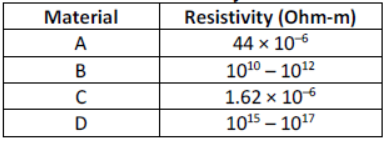Which material would you suggest to be used in electric heating devices? Give reason for your choice.
Answer: (a) The SI unit of current is Ampere. Current (I) can be expressed in terms of the charge (Q) flowing in time (t) as: I = Q /t
(b) As materials used in electric heating devices are generally made up of alloys having resistivity in the range of 10–8 Ohm m to 10–6 Ohm–m, material A will be used for electric
heating devices as it’s resistivity is more than that of C, which is a metal since its resistivity is very low. B and D are insulators as they have very high resistivity.

OR

(a) On what factors does the resistance of a conductor depend?
(b) Calculate the resistance of an aluminium cable of length 10 km and diameter 2.0 mm if the resistivity of aluminium is 2.7 × 10–8 Ωm.
Answer: (a) Resistance of a conductor depends on the following factors:
(1) Length of the conductor
(2) Area of cross section of the conductor
(3) Nature of material of the conductor
(4) Temperature of the conductor
(b) Given: l = 10 km = 10000 m; d = 2 mm; r = 1 mm = 10–3 m; r = 2.7 × 10–8 Ω m24. What is a rainbow? Draw a well labelled diagram to show the formation of a rainbow.
Answer: Rainbow - A natural spectrum of sunlight appearing in the sky after a rain shower.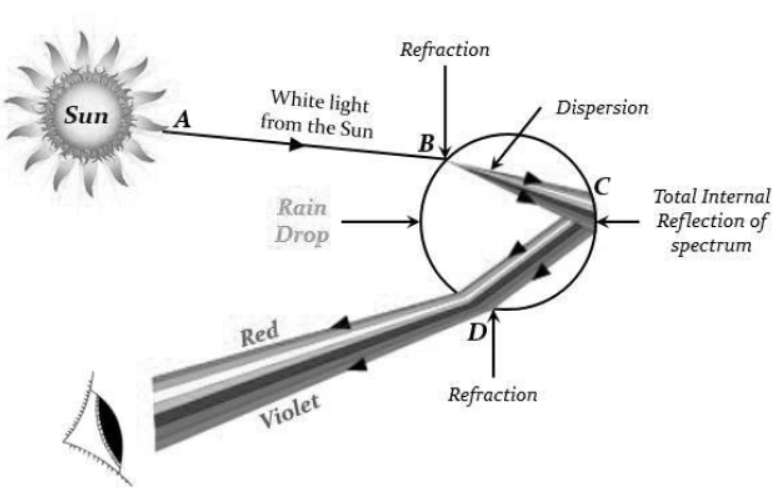25. What are the differences between the transport of materials in xylem and phloem?

 S. No. Xylem Phloem 1 Xylem conducts water and dissolvedminerals from roots to leaves and otherparts. Phloem conducts prepared food materialfrom leaves to other parts of plant indissolved form. 2 In xylem, transport of material takesplace through vessels and tracheids. In phloem, transport of material takes placewith the help of companion cells. 3 In xylem, upward movement of waterand dissolved materials is mainlyachieved by transpiration pull. It iscaused due to sanction created byevaporation of water molecules from thestomata of a leaf. In translocation, material is transferred intophloem tissue using energy from ATP.This increases the osmotic pressure thatmoves the material in the phloem to thetissues which have less pressure. 4 Movement of water is achieved bysimple physical forces. There is noexpenditure of energy. So, ATPmolecules are not required. The translocation in phloem is an activeprocess and requires energy. This energy istaken from ATP molecules.

26. Give reasons for the following observations:
(a) Covalent compounds are poor conductors of electricity.
(b) Highly reactive metals cannot be obtained from their oxides by heating them with carbon.
Answer: It is because covalent compounds do not form ions.
(b) It is because these metal, themselves are strong reducing agents. Therefore, cannot be reduced by reducing agent like carbon.

SECTION – C

27. Give reasons for the following:
(a) Ionic compounds have high melting and boiling point
(b) Ionic compounds conduct electricity in molten state
(c) Ionic compounds are solid at room temperature and are somewhat hard.
Answer: (a) Ionic compounds have high boiling and melting point due to the presence of strong bond between cations and anions. To break or overcome these strong forces of attraction, a large amount of energy is required.
(b) Ionic compounds conduct electricity in molten state. Conductivity depends on the presence of number of free ions. Solid ionic compounds cannot conduct electricity because of the absence of ions (electrons) in the crystal structure. When the ionic compound is present in molten state, crystal structure deforms and they can easily conduct electricity with the free ions.
(c) Ionic compounds are solid at room temperature and are somewhat hard. Due to the presence of strong force of attraction between the positive and negative ions a solid ionic compound formed becomes hard and solid at room temperature.

28. (a) What is a solenoid?
(b) Draw the pattern of magnetic field lines of (i) a current carrying solenoid and (ii) a bar magnet.
Answer: (a) A coil of many turns of insulated copper wire wrapped closely in the shape of a cylinder is called solenoid.
(b)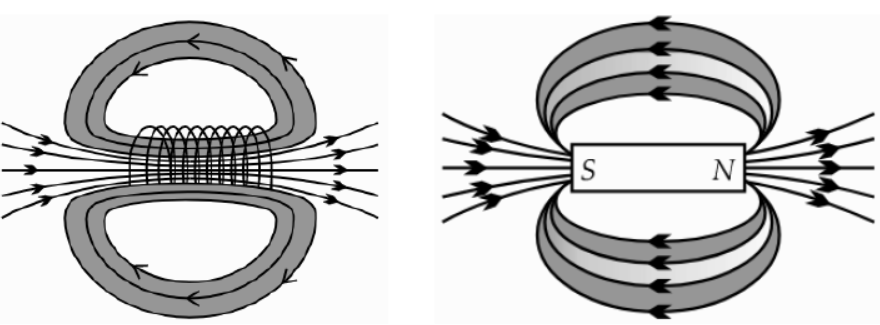OR

Suppose your parents have constructed a two room house and you want that in the living room there should be a provision of one electric bulb, one electric fan, a refrigerator and a plug point for appliances of power upto 2 kilowatt. Draw a circuit diagram showing electric fuse and earthing as safety devices.(i) Four components should be labelled.
(ii) All of them should be in parallel and there should be a fuse for safety.
(iii) Live and earth wires should be there.

29. Sahil took five solutions A, B, C, D and E and tested with universal indicator showed pH as 4, 1, 11, 7 and 9 respectively. Which solution is: (a) Neutral (b) Strongly alkaline (c) Strongly acidic (d) Weakly acidic (E) Weakly alkaline?
Arrange the pH in increasing order of hydrogen ion concentration.
Answer: Given pH for the solutions are A = 4, B = 1, C = 11, D = 7, E = 9.
Hydrogen ions concentration increases with decrease in pH value and thus strength of acid increases with decrease in pH value from 7 to 0. On the other hand, hydroxide ion’s
concentration decreases with increase in pH value and thus strength of bases increases with increase in pH value from 7 to 14. While neutral solution has pH value = 7.
Therefore, (a) Solution D is neutral having pH value equal to 7.
(b) Solution C is strongly alkaline as its pH value is equal to 11
(c) Solution B is strongly acidic as its pH value is equal to 1
(d) Solution A is weakly acidic as its pH value is equal to 4
(e) Solution E is weakly alkaline as its pH value is equal to 9
Hence arrangement of given pH value in increasing order of hydrogen ion concentration: C
(11) < E (9) < D (7) < A (4) < B (1)

30. (a) Describe how a squirrel uses its hormonal system to react to a dangerous situation.
(b) How do sensory and motor neurons differ from one another?
Answer: (a) The hormone adrenaline is released into a squirrel's blood when it detects danger, increasing heart rate and blood flow to tissues. As a result, its cells and tissues receive energy more quickly, allowing it to flee dangerous situations.
(b) Sensory neurons transmit impulses to the central nervous system after receiving information from receptors. In order for a muscle, gland, or organ to respond, motor neurons carry messages from the control nervous system to those tissues.